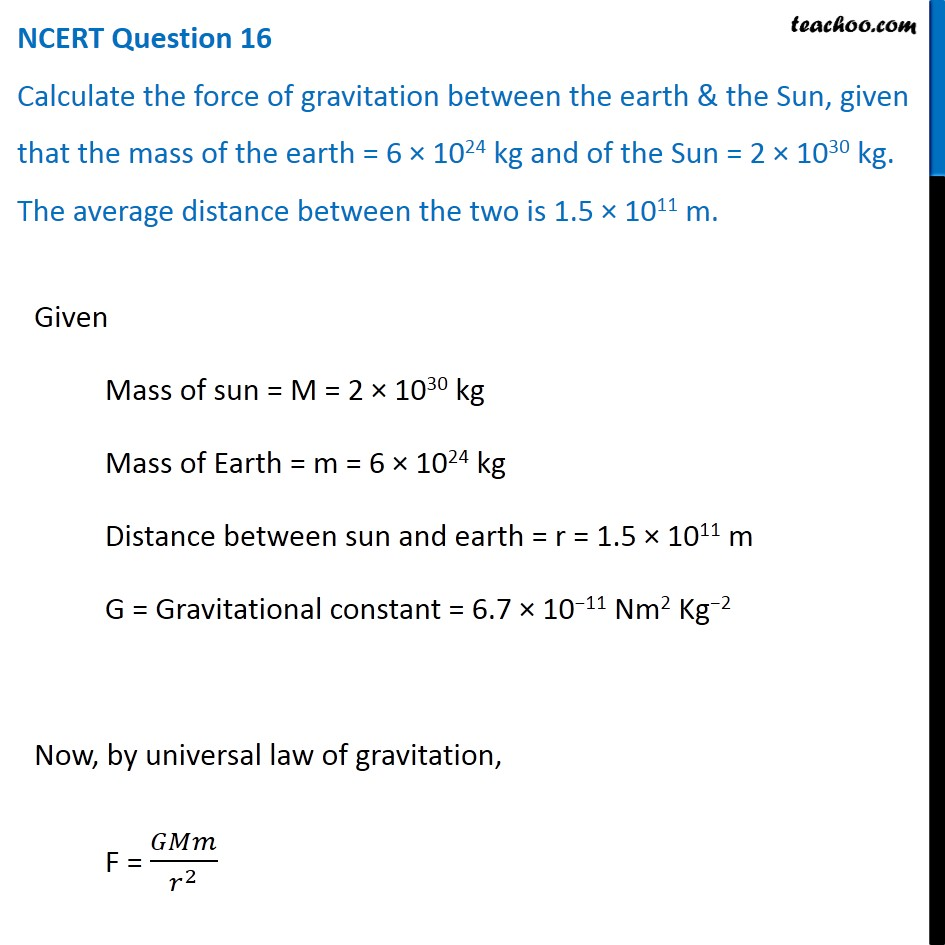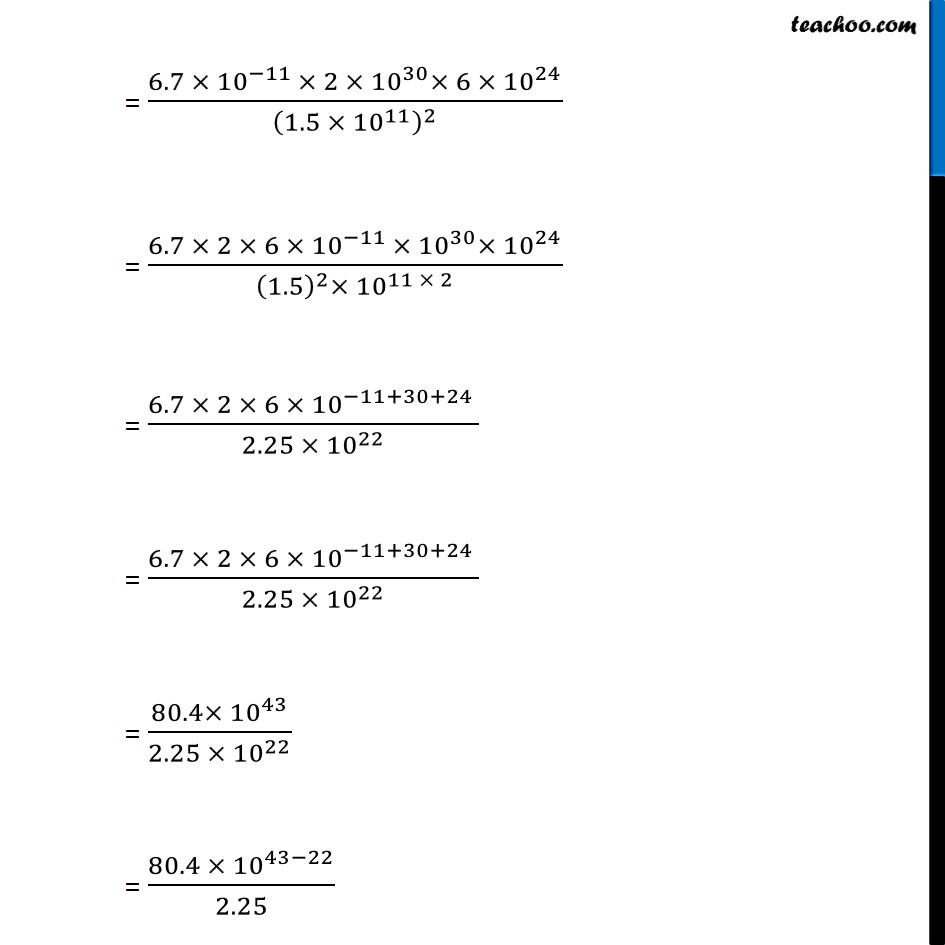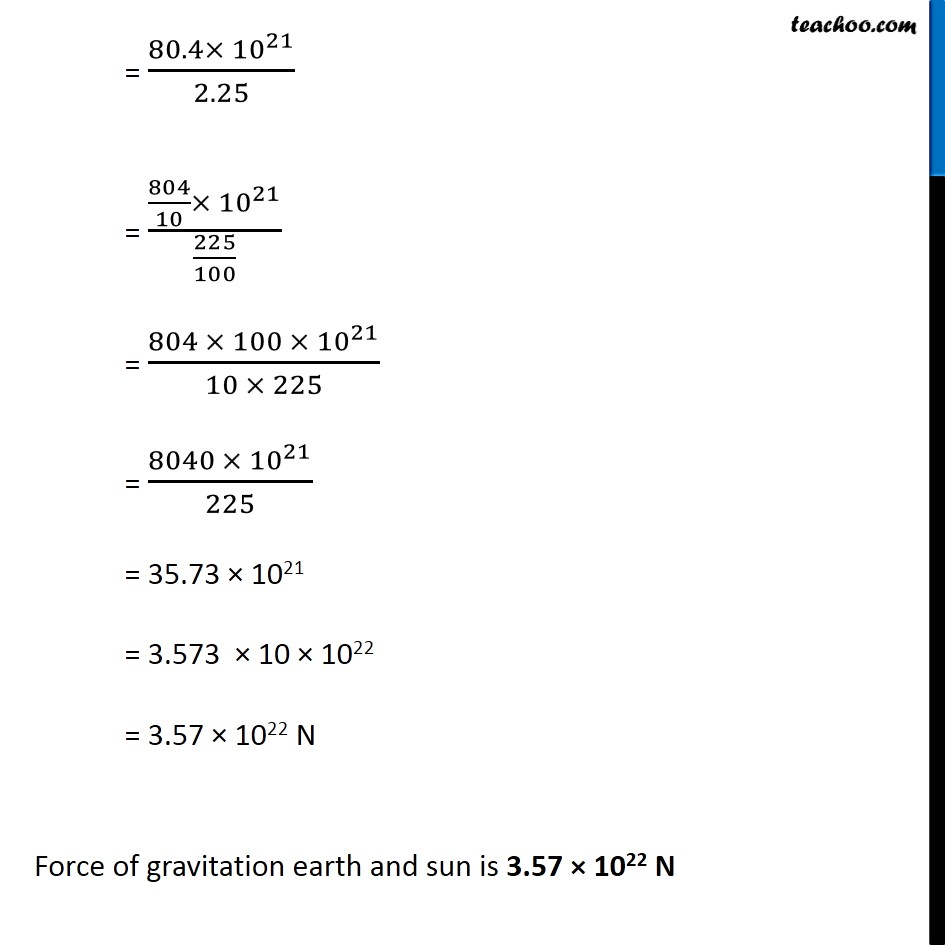NCERT Questions

Class 9
Chapter 10 Class 9 - GravitationLearn in your speed, with individual attention - Teachoo Maths 1-on-1 Class

### Transcript

NCERT Question 16 Calculate the force of gravitation between the earth & the Sun, given that the mass of the earth = 6 × 1024 kg and of the Sun = 2 × 1030 kg. The average distance between the two is 1.5 × 1011 m. Given Mass of sun = M = 2 × 1030 kg Mass of Earth = m = 6 × 1024 kg Distance between sun and earth = r = 1.5 × 1011 m G = Gravitational constant = 6.7 × 10−11 Nm2 Kg−2 Now, by universal law of gravitation, F = 𝐺𝑀𝑚/𝑟^2 = (6.7 ×〖 10〗^(−11 )× 2 ×〖 10〗^30× 6 × 〖10〗^24)/(1.5 × 〖10〗^11 )^2 = (6.7 × 2 × 6 × 〖10〗^(−11 )×〖 10〗^30× 〖10〗^24)/((1.5)^2× 〖10〗^(11 × 2) ) = (6.7 × 2 × 6 × 〖10〗^(−11+30+24 ))/(2.25 × 〖10〗^22 ) = (6.7 × 2 × 6 × 〖10〗^(−11+30+24 ))/(2.25 × 〖10〗^22 ) = (80.4×〖 10〗^43)/(2.25 × 〖10〗^22 ) = (80.4 ×〖 10〗^(43−22))/2.25 = (6.7 ×〖 10〗^(−11 )× 2 ×〖 10〗^30× 6 × 〖10〗^24)/(1.5 × 〖10〗^11 )^2 = (6.7 × 2 × 6 × 〖10〗^(−11 )×〖 10〗^30× 〖10〗^24)/((1.5)^2× 〖10〗^(11 × 2) ) = (6.7 × 2 × 6 × 〖10〗^(−11+30+24 ))/(2.25 × 〖10〗^22 ) = (6.7 × 2 × 6 × 〖10〗^(−11+30+24 ))/(2.25 × 〖10〗^22 ) = (80.4×〖 10〗^43)/(2.25 × 〖10〗^22 ) = (80.4 ×〖 10〗^(43−22))/2.25